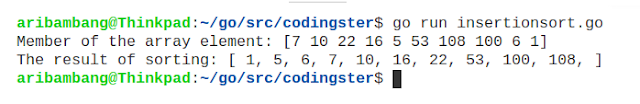-->

## Social Items

In this post, we will learn to create an insertion sort algorithm program in the Go programming language (Golang). We try to sort 10 numbers randomly, with numbers that will be sorted as follows: 7, 10, 22, 16, 5, 53, 108, 100, 6, 1.

### Source Code :

``````package main

import "fmt"

func insertionSort(arr []int) {

for i := 0; i < len(arr); i++ {
tmp := arr[i]
j := i
for j > 0 && arr[j-1] > tmp {
arr[j] = arr[j-1]
j--
}
arr[j] = tmp
}
}

func main() {
arr := []int{7, 10, 22, 16, 5, 53, 108, 100, 6, 1}
fmt.Print("Member of the array element: ", arr, "\n", "The result of sorting: [ ")
insertionSort(arr)
for i := 0; i < len(arr); i++ {
fmt.Print(arr[i], ", ")
}
fmt.Print("]\n")
}
``````

Save the source code with the name of insertionsort.go, don't forget the extension should .go

### Compile & Run :

Here's how to compile source code manually:
``````
\$ go build insertionsort.go
\$ ./insertionsort
``````
You can run without having to compile it:
``````
\$ go run insertionsort.go
``````

### The Output of Program :Picture of the result insertion sort algorithm## Insertion Sort Algorithm In Go (Golang)

In this post, we will learn to create an insertion sort algorithm program in the Go programming language (Golang). We try to sort 10 numbers randomly, with numbers that will be sorted as follows: 7, 10, 22, 16, 5, 53, 108, 100, 6, 1.

### Source Code :

``````package main

import "fmt"

func insertionSort(arr []int) {

for i := 0; i < len(arr); i++ {
tmp := arr[i]
j := i
for j > 0 && arr[j-1] > tmp {
arr[j] = arr[j-1]
j--
}
arr[j] = tmp
}
}

func main() {
arr := []int{7, 10, 22, 16, 5, 53, 108, 100, 6, 1}
fmt.Print("Member of the array element: ", arr, "\n", "The result of sorting: [ ")
insertionSort(arr)
for i := 0; i < len(arr); i++ {
fmt.Print(arr[i], ", ")
}
fmt.Print("]\n")
}
``````

Save the source code with the name of insertionsort.go, don't forget the extension should .go

### Compile & Run :

Here's how to compile source code manually:
``````
\$ go build insertionsort.go
\$ ./insertionsort
``````
You can run without having to compile it:
``````
\$ go run insertionsort.go
``````

### The Output of Program :Picture of the result insertion sort algorithm